# Revision Notes: Heat Transfer Notes | Study Mock Test Series for JEE Main & Advanced - JEE

## JEE: Revision Notes: Heat Transfer Notes | Study Mock Test Series for JEE Main & Advanced - JEE

The document Revision Notes: Heat Transfer Notes | Study Mock Test Series for JEE Main & Advanced - JEE is a part of the JEE Course Mock Test Series for JEE Main & Advanced.
All you need of JEE at this link: JEE

What is Heat Transfer?

The movement of heat across the border of the system due to a difference in temperature between the system and its surroundings.

Interestingly, the difference in temperature is said to be a ‘potential’ that causes the transfer of heat from one point to another. Besides, heat is also known as flux.

How is Heat Transferred?

Heat can travel from one place to another in several ways. The different modes of heat transfer include:

• Conduction
• Convection

Meanwhile, if the temperature difference exists between the two systems, heat will find a way to transfer from the higher to the lower system.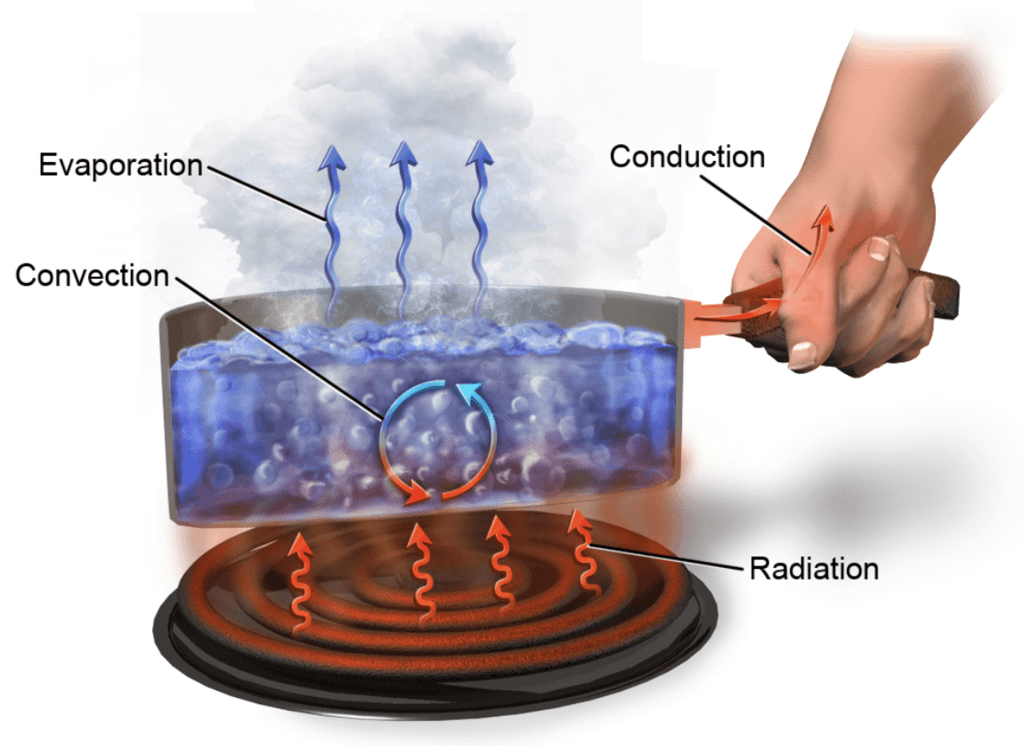What is Conduction?

The process in which heat flows from objects with higher temperature to objects with lower temperature.

Following are the examples of conduction:

1. Ironing of clothes is an example of conduction where the heat is conducted from the iron to the clothes.
2. Heat is transferred from hands to ice cube resulting in the melting of an ice cube when held in hands.
3. Heat conduction through the sand at the beaches. This can be experienced during summers. Sand is a good conductor of heat.

Conduction Equation

The coefficient of thermal conductivity shows that a metal body conducts heat better when it comes to conduction. The rate of conduction can be calculated by the following equation: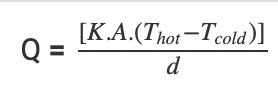Where,

• Q is the transfer of heat per unit time
• K is the thermal conductivity of the body
• A is the area of heat transfer
• Thot is the temperature of the hot region
• Tcold is the temperature of the cold region
• d is the thickness of the body

What is Convection?

The movement of fluid molecules from higher temperature regions to lower temperature regions.

Examples of convection include:

1. The boiling of water, that is molecules that are denser move at the bottom while the molecules which are less dense move upwards resulting in the circular motion of the molecules so that water gets heated.
2. Warm water around the equator moves towards the poles while cooler water at the poles moves towards the equator.
3. Blood circulation in warm-blooded animals takes place with the help of convection, thereby regulating the body temperature.

Convection Equation

As the temperature of the liquid increases, the liquid’s volume also has to increase by the same factor and this effect is known as displacement. The equation to calculate the rate of convection is as follows: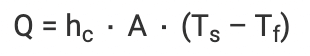Where,

• Q is the heat transferred per unit time
• Hc is the coefficient of convective heat transfer
• A is the area of heat transfer
• Ts is the surface temperature
• Tf is the fluid temperature

It is defined as that mode of transmission of heat in which heat travels from hot body to cold body in straight lines without heating the intervening medium.

Following are the examples of radiation:

1. Microwave radiation emitted in the oven is an example of radiation.
2. UV rays coming from the sun is an example of radiation.
3. The release of alpha particles during the decaying of Uranium-238 into Thorium-234 is an example of radiation.

As temperature rises, the wavelengths in the spectra of the radiation emitted decreases and shorter wavelengths radiations are emitted. Thermal radiation can be calculated by Stefan-Boltzmann law: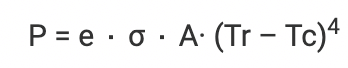Where,

• P is the net power of radiation
• A is the area of radiation
• Tr is the radiator temperature
• Tc is the surrounding temperature
• e is emissivity and σ is Stefan’s constant

Try yourself:When water is kept in a very hot pan, the entire water heats up. Which mode of heat transfer is responsible for this?

What is Thermal Conductivity?

Thermal conductivity refers to the ability of a given material to conduct/transfer heat. It is generally denoted by the symbol ‘k’ but can also be denoted by ‘λ’ and ‘κ’.

The thermal conductivity of a material is described by the following formula: K = (QL)/(AΔT)

Where,

• K is the thermal conductivity in W/m.K
• Q is the amount of heat transferred through the material in Joules/second or Watts
• L is the distance between the two isothermal planes
• A is the area of the surface in square meters
• ΔT is the difference in temperature in Kelvin

Coefficient of Thermal Conductivity

The coefficient of thermal conductivity of the material of a rod is defined as the heat current (amount of heat flowing per second) flowing per unit area between two cross-section of the rod each of area 1 m2 and separated 1 m apart.

Rate of flow of heat = dQ/dt

Heat current, through a conducting rod, is defined as the amount of heat conducted across any cross-section of the rod in one second.

H= dQ/dt

H depends upon the following factors:

1. Area of a cross-section of rod: H ∝ A
3. Nature of the material
So, H = -KA (dθ/dx)
Here K is called the coefficient of thermal conductivity of the material of rod. It depends upon the nature of the material of the rod.
4. The total heat Q crossing from one cross-section to the other in time t:
Q = KA(θ12)t/l
Or K = Ql/A(θ12)t

Dimension of K: [K] = [M1L1T-3K-1]
Unit: C.G.S- cal cm-1s-1 ºC-1
S.I.: Wm-1K-1

It is defined as the rate of change of temperature of the different cross-section with distance.

Thermal Conductance (σh)

It is defined as heat current per unit temperature difference.

σh= KA/l
σh= H/dθ
Unit- S.I- WK-1

Thermal Resistance (RH)

The thermal resistance, of a conductor, is defined as the temperature difference between its two cross-sections when a unit heat current flows through it.

Reciprocal thermal conductance is known as the thermal resistance of the substance.

Rh= 1/σH = l/KA = dθ/H

Units of Rh: S.I – W-1K

Thermal resistance of a conductor of length d:  RTH = d/KA

Try yourself:Select the correct statement.

Flow of heat through a composite slab

1. Thermal resistance in series: Thermal resistance of the composite slab is equal to the sum of their individual thermal resistances.
(l1 +l2)/KA = (l1/K1A) + (l2/K2A)
Rcomb = R+ Rh'
If l1= l= l, then, K = 2K1K2/K1+K2
Temperature of the interface:
θ= [θ1Rh' + θ2Rh]/ [R+ Rh'] or
θ= [θ1K1l+ θ2 K2l1]/ [K1l+ K2l1]
2. Thermal resistance in parallel: Reciprocal of the combined thermal resistance is equal to the sum of the reciprocals of individual thermal resistances.
1/Rcomb = 1/Rh + 1/Rh

Bolometer

A bolometer is a device for measuring the power of incident electromagnetic radiation via the heating of a material with a temperature-dependent electrical resistance.

If Rt and R0 are the resistances of the conductor at 0ºC and tºC, then, Rt = R0(1+αt), Here α is the temperature coefficient of change of resistance with temperature.

Absorptive power (a)

Absorptive power (a) of the substance is defined as the ratio between amounts of heat absorbed by it to the total amount of heat incident upon it.Reflecting Power (r)

Reflecting power (r) of a substance is defined as the ratio between the amount of heat reflected by the substance to the total amount of heat incident upon it.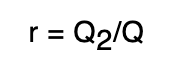Transmitting power (t)

Transmitting power (t) of a substance is defined as the ratio between the amount of heat transmitted by the body to the total amount of heat incident upon it.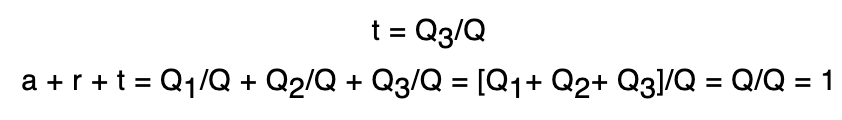Radiant emittance of a body at a temperature T is defined as the total amount of energy (for all wavelengths) radiated per unit time, per unit area by the body.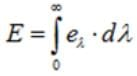Unit - S.I: Jm-2s-1
C.G.S: erg cm-2s-1

Energy Density

Total energy density (U) at any point is defined as the radiant energy per unit volume, around that point, for wave-lengths taken together.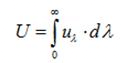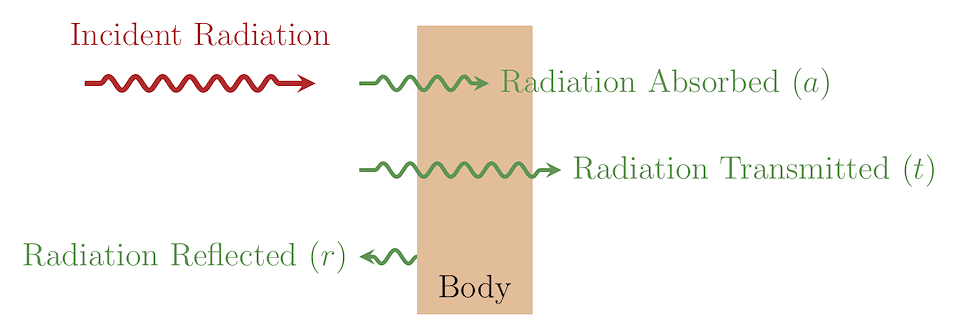It states that at any temperature, the ratio of emissive power eλ of a body to its absorptive power aλ, for a particular wave-length, is always constant and is equal to the emissive power of perfect black body for that wavelength.

eλ/aλ = Constant = Eλ

This implies the ratio between eλ and aλ for anybody is a constant quantity (= Eλ).

Wien’s Displacement Law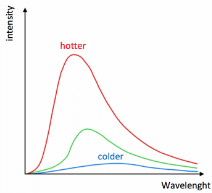It states that wavelength of radiation which is emitted with maximum intensity varies inversely as the absolute temperature of the body.
λm×T = Constant

Stefan’s Law

Radiant emittance or the energy radiated per second per unit area by a perfect black body varies directly as the fourth power of its absolute temperature.

E = σT4

Here σ is the Stefan’s constant and its value is 5.735×10-8 Wm-2K-4

Spectral emissive power

Spectral emissive power is defined as the rate at which radiation of wavelength l is emitted in all directions form a surface per unit wavelength dl about l.

eλ= Q/At(dλ)

Emissivity

Emissivity is defined as the ratio of the energy radiated from a material's surface to that radiated from a a perfect emitter, known as a blackbody, at the same temperature and wavelength and under the same viewing conditions.

ε = e/E,  0 ≤ ε ≤1

• The emissivity of a body is numerically equal to its absorptive power.
e = aλ
• Emissivity of the body determines the radiant emittance of a body.
• Emissivity of a perfect body is always one,
• Emissivity of anybody other than a perfectly black body is less than one.
• Emissivity of anybody is numerically equal to its absorbing power.

Rate of loss of heat: -dQ/dT = εAσ(θ4- θ04)

For spherical objects: (dQ/dT)1/(dQ/dT)2 = r12/r22

Newton’s Law of Colling

It states that the rate of loss of heat of a body is directly proportional to the temperature difference between the body and surroundings.
dQ/dt = -K(T-T0) or (T-T0) ∝ e-KT

Wien's displacement law states that the black body radiation curve for different temperatures peaks at a wavelength inversely proportional to the temperature.

Eλdλ = (A/λ5) f(λT) dλ = (A/λ5) e-a/λT

Solar Constant

Solar constant, the total radiation energy received from the Sun per unit of time per unit of area on a theoretical surface perpendicular to the Sun’s rays and at Earth’s mean distance from the Sun.

S = (RS/RES)σT4

Try yourself:According to Newton’s Law of Colling,

The rate of cooling of water increases with a fall in temperature.

The document Revision Notes: Heat Transfer Notes | Study Mock Test Series for JEE Main & Advanced - JEE is a part of the JEE Course Mock Test Series for JEE Main & Advanced.
All you need of JEE at this link: JEEUse Code STAYHOME200 and get INR 200 additional OFF

## Mock Test Series for JEE Main & Advanced

2 videos|324 docs|160 tests

Track your progress, build streaks, highlight & save important lessons and more!

,

,

,

,

,

,

,

,

,

,

,

,

,

,

,

,

,

,

,

,

,

;